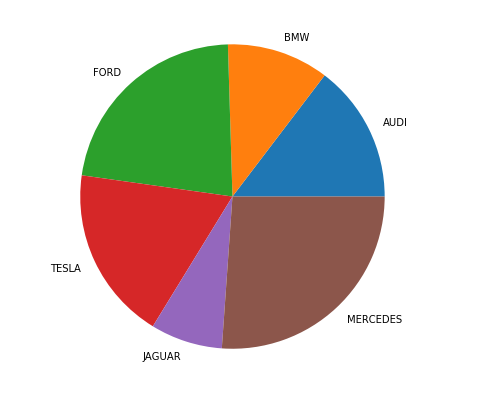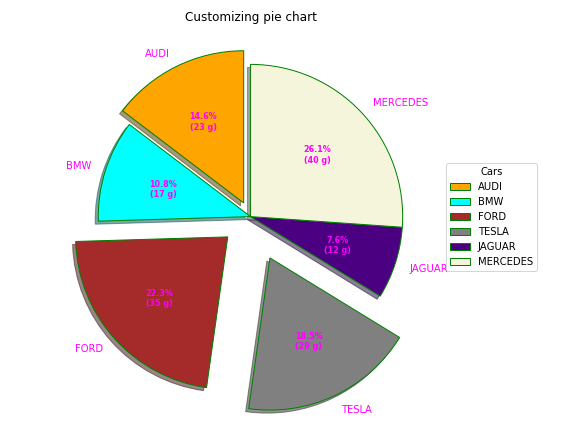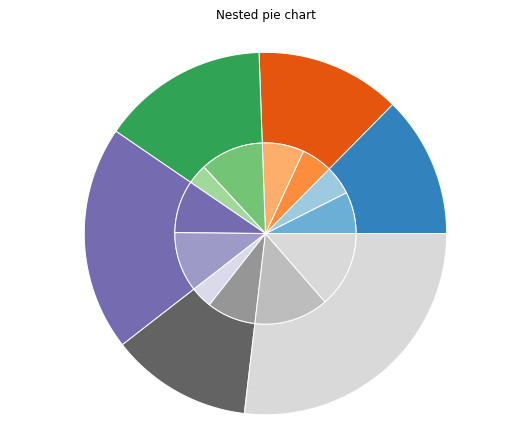Open in App
Not now

# Plot a pie chart in Python using Matplotlib

• Difficulty Level : Medium
• Last Updated : 30 Nov, 2021

A Pie Chart is a circular statistical plot that can display only one series of data. The area of the chart is the total percentage of the given data. The area of slices of the pie represents the percentage of the parts of the data. The slices of pie are called wedges. The area of the wedge is determined by the length of the arc of the wedge. The area of a wedge represents the relative percentage of that part with respect to whole data. Pie charts are commonly used in business presentations like sales, operations, survey results, resources, etc as they provide a quick summary.

## Creating Pie Chart

Matplotlib API has pie() function in its pyplot module which create a pie chart representing the data in an array.

Syntax: matplotlib.pyplot.pie(data, explode=None, labels=None, colors=None, autopct=None, shadow=False)
Parameters:
data represents the array of data values to be plotted, the fractional area of each slice is represented by data/sum(data). If sum(data)<1, then the data values returns the fractional area directly, thus resulting pie will have empty wedge of size 1-sum(data).
labels is a list of sequence of strings which sets the label of each wedge.
color attribute is used to provide color to the wedges.
autopct is a string used to label the wedge with their numerical value.

Let’s create a simple pie chart using the pie() function:
Example:

## Python3

 `# Import libraries` `from` `matplotlib ``import` `pyplot as plt` `import` `numpy as np`     `# Creating dataset` `cars ``=` `[``'AUDI'``, ``'BMW'``, ``'FORD'``,` `        ``'TESLA'``, ``'JAGUAR'``, ``'MERCEDES'``]`   `data ``=` `[``23``, ``17``, ``35``, ``29``, ``12``, ``41``]`   `# Creating plot` `fig ``=` `plt.figure(figsize ``=``(``10``, ``7``))` `plt.pie(data, labels ``=` `cars)`   `# show plot` `plt.show()`

Output:## Customizing Pie Chart

A pie chart can be customized on the basis several aspects. The startangle attribute rotates the plot by the specified degrees in counter clockwise direction performed on x-axis of pie chart. shadow attribute accepts boolean value, if its true then shadow will appear below the rim of pie. Wedges of the pie can be customized using wedgeprop which takes Python dictionary as parameter with name values pairs denoting the wedge properties like linewidth, edgecolor, etc. By setting frame=True axes frame is drawn around the pie chart.autopct controls how the percentages are displayed on the wedges. Let us try to modify the above plot:
Example 1:

## Python3

 `# Import libraries` `import` `numpy as np` `import` `matplotlib.pyplot as plt`     `# Creating dataset` `cars ``=` `[``'AUDI'``, ``'BMW'``, ``'FORD'``, ` `        ``'TESLA'``, ``'JAGUAR'``, ``'MERCEDES'``]`   `data ``=` `[``23``, ``17``, ``35``, ``29``, ``12``, ``41``]`     `# Creating explode data` `explode ``=` `(``0.1``, ``0.0``, ``0.2``, ``0.3``, ``0.0``, ``0.0``)`   `# Creating color parameters` `colors ``=` `( ``"orange"``, ``"cyan"``, ``"brown"``,` `          ``"grey"``, ``"indigo"``, ``"beige"``)`   `# Wedge properties` `wp ``=` `{ ``'linewidth'` `: ``1``, ``'edgecolor'` `: ``"green"` `}`   `# Creating autocpt arguments` `def` `func(pct, allvalues):` `    ``absolute ``=` `int``(pct ``/` `100.``*``np.``sum``(allvalues))` `    ``return` `"{:.1f}%\n({:d} g)"``.``format``(pct, absolute)`   `# Creating plot` `fig, ax ``=` `plt.subplots(figsize ``=``(``10``, ``7``))` `wedges, texts, autotexts ``=` `ax.pie(data, ` `                                  ``autopct ``=` `lambda` `pct: func(pct, data),` `                                  ``explode ``=` `explode, ` `                                  ``labels ``=` `cars,` `                                  ``shadow ``=` `True``,` `                                  ``colors ``=` `colors,` `                                  ``startangle ``=` `90``,` `                                  ``wedgeprops ``=` `wp,` `                                  ``textprops ``=` `dict``(color ``=``"magenta"``))`   `# Adding legend` `ax.legend(wedges, cars,` `          ``title ``=``"Cars"``,` `          ``loc ``=``"center left"``,` `          ``bbox_to_anchor ``=``(``1``, ``0``, ``0.5``, ``1``))`   `plt.setp(autotexts, size ``=` `8``, weight ``=``"bold"``)` `ax.set_title(``"Customizing pie chart"``)`   `# show plot` `plt.show()`

Output:Example 2: Creating a Nested Pie Chart

## Python3

 `# Import libraries` `from` `matplotlib ``import` `pyplot as plt` `import` `numpy as np`     `# Creating dataset` `size ``=` `6` `cars ``=` `[``'AUDI'``, ``'BMW'``, ``'FORD'``, ` `        ``'TESLA'``, ``'JAGUAR'``, ``'MERCEDES'``]`   `data ``=` `np.array([[``23``, ``16``], [``17``, ``23``],` `                 ``[``35``, ``11``], [``29``, ``33``],` `                 ``[``12``, ``27``], [``41``, ``42``]])`   `# normalizing data to 2 pi` `norm ``=` `data ``/` `np.``sum``(data)``*``2` `*` `np.pi`   `# obtaining ordinates of bar edges` `left ``=` `np.cumsum(np.append(``0``, ` `                           ``norm.flatten()[:``-``1``])).reshape(data.shape)`   `# Creating color scale` `cmap ``=` `plt.get_cmap(``"tab20c"``)` `outer_colors ``=` `cmap(np.arange(``6``)``*``4``)` `inner_colors ``=` `cmap(np.array([``1``, ``2``, ``5``, ``6``, ``9``,` `                              ``10``, ``12``, ``13``, ``15``,` `                              ``17``, ``18``, ``20` `]))`   `# Creating plot` `fig, ax ``=` `plt.subplots(figsize ``=``(``10``, ``7``),` `                       ``subplot_kw ``=` `dict``(polar ``=` `True``))`   `ax.bar(x ``=` `left[:, ``0``],` `       ``width ``=` `norm.``sum``(axis ``=` `1``),` `       ``bottom ``=` `1``-``size,` `       ``height ``=` `size,` `       ``color ``=` `outer_colors, ` `       ``edgecolor ``=``'w'``,` `       ``linewidth ``=` `1``,` `       ``align ``=``"edge"``)`   `ax.bar(x ``=` `left.flatten(),` `       ``width ``=` `norm.flatten(),` `       ``bottom ``=` `1``-``2` `*` `size,` `       ``height ``=` `size,` `       ``color ``=` `inner_colors,` `       ``edgecolor ``=``'w'``,` `       ``linewidth ``=` `1``,` `       ``align ``=``"edge"``)`   `ax.``set``(title ``=``"Nested pie chart"``)` `ax.set_axis_off()`   `# show plot` `plt.show()`

Output:My Personal Notes arrow_drop_up
Related Articles